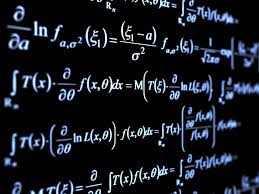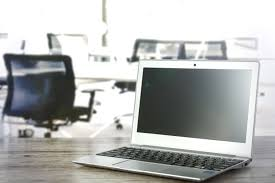## Posts

Showing posts with the label ALGEBRA

### EXPANSION SLOTS IN MOTHERBOARDEXPANSION SLOTS IN MOTHERBOARD  Friends today i am going to share my knowledge and understanding of the expansion slots. So lets begin with our topic. Expansion slots are used to provide additional properties for carrying the computation task such as additional video, audio and sound, advanced graphics and Ethernet.   So lets begin with our knowledge hunting. I will start by AGP expansion slots. AGP AGP stands for ACCELARATED GRAPHICS PORT.  AGP was introduced with high speed 3D graphics display in 1996.  It is used for older graphics card types which is discontinued by PCI EX16 graphics port in 2005.   These were kernel version of AGP most of the brand in 1.5 volt DC.  AGP 1x channel and 66MHZ clock speed resulting a data table of 266 MBPS.   AGP 2x, 4x ,8x specification multiply MHZ clock to produce increase throughput. AGP 8x produces effective clock frequency of 533 MHZ resulting a throughput of 2 GBPS (2133MHZ) resulting a throughput of over a 32 bit channel. PCI PCI  stands for P

### ARITHMETIC CALCULATION IN COMPUTERSARITHMETIC OPERATION AND CONVERSION DONE BY COMPUTER As we have seen in previous post that arithmetic computation is an important thing in calculation, if a person doesn’t know arithmetic computations,   he will be doomed to be in trouble during calculations that happens   in everyday life.   So it is necessary to learn arithmetic computation.   So in this post I am going to take one step further.   I am going to write about octal and hexadecimal numbers. Now let us discuss some arithmetic conversion.   Let us see what is octal and hexadecimal numbers used by digital computer. The conversion from and to binary, octal and hexadecimal plays an important part in digital computers.   Since 2 3 = 8 and 2 4 = 16 each octal digit corresponds to three binary digits and each four binary digit corresponds to one hexadecimal digit.   The conversion of from binary to octal is easily accomplished by partitioning the binary into group of three digit each, starting from binary

### MATHEMATICS AND COMPUTER SCIENCE RELATIONSHIPMATHEMATICS AND COMPUTER SCIENCE RELATIONSHIP. In today’s world mathematics is a must learned subject .   In all the inventions and discovery , the main reason for their success of the practical’s are due to mathematics.   So in order to make a career in today’s world require mathematics.   But this Is blog of computers science and information technology, then why should we read mathematics so it requires mathematics to understand the reason behind such technology.   So I am going to write something about mathematics for its uses and technology in the computer world. One of the main aims of logic is to provide rules by which one can determine whether any particular argument or reasoning is valid (correct) Logic is concerned with all kinds of reasoning whether they be legal arguments or mathematical proofs or conclusions in a scientific theory based upon a set of hypothesis.   Because of the diversity of their application these rules are called rules of inference,

### IS DIGITAL COMPUTERS ARE VALUABLE?DIGITAL COMPUTERS Today computers are used extensively in every day life.  It is used in basic as well as industrial, scientific and commercial purposes.  Our space program would not have been well developed without the real time monitoring of the machine of the spacecraft which was going to land on moon or any other planet of the scheduled program or launching of satellites on the orbit of earth. This is the scientific purpose i told you about while it has commercial purposes.  Like automatic data processing which the business enterprise uses. It has many works in educational purposes for example multiple choice question answers can be checked without any error and then the results can be printed out automatically with the help of the printer which is connected to the digital computer.   Digital computer are also used in air traffic control to control the aviation industry to help them control the planes by sending them data about the distance between the planes and t

### LOGIC OF COMPUTERSLOGIC OF COMPUTER IN PAPER AS WELL AS PRACTICAL USE We always use logic in every day matters, so do the machines.   But we were taught by experiences we count and the people we trust and these things matters most because it will help in dark times.   But to make the machine understand the logic is very hefty work. But this is certainly not impossible.   In order to understand logic we use integrated circuits.   But to do this we need to design the integrated circuits on paper.   This requires certain rules of algebra.   This I am going talk about the rule of an algebra called the duality principal. It states that every algebraic expression deducible from the postulates of Boolean algebra remains valid if the operators and identity elements are interchanged.   In a two value Boolean algebra, the identity elements and the elements of the set B are same 1 and 0.   The duality principal has many applications.   If the dual of an algebraic expression is desired, we simply

### THEORITICAL ALGEBRA IN COMPUTERSALGEBRA IN COMPUTERS Algebra is a subject in which the problem of the sum is solved with the help of letters (I mean the letters of English language as well as Greek language).  It is mostly used by the mathematicians, engineers, scientist as well as businessmen to solve any problem of the world.  Algebra is very important in many science as well as commerce studies.  So we are going to dig deep in algebra used in computers. We will consider Boolean algebra as it is used by the computers to solve real life problems.  So let us begin. Boolean algebra, like any other     mathematical system , may be with a set of elements , a set of operators and a number of unproved axioms or postulates.    A set of elements is any collection of objects having a common property.  If S is a set and x is an element of that set the x€ S denotes that x is an element of that set.   A set with de-numberable (means a small number) number of elements is specified by braces:  A={1, 2, 3, 4} tha